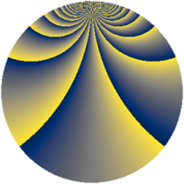# Properties

 Label 475.2.bcLevel $475$ Weight $2$ Character orbit 475.bc Rep. character $\chi_{475}(6,\cdot)$ Character field $\Q(\zeta_{45})$ Dimension $1152$ Newform subspaces $1$ Sturm bound $100$ Trace bound $0$

# Related objects

## Defining parameters

 Level: $$N$$ $$=$$ $$475 = 5^{2} \cdot 19$$ Weight: $$k$$ $$=$$ $$2$$ Character orbit: $$[\chi]$$ $$=$$ 475.bc (of order $$45$$ and degree $$24$$) Character conductor: $$\operatorname{cond}(\chi)$$ $$=$$ $$475$$ Character field: $$\Q(\zeta_{45})$$ Newform subspaces: $$1$$ Sturm bound: $$100$$ Trace bound: $$0$$

## Dimensions

The following table gives the dimensions of various subspaces of $$M_{2}(475, [\chi])$$.

Total New Old
Modular forms 1248 1248 0
Cusp forms 1152 1152 0
Eisenstein series 96 96 0

## Trace form

 $$1152 q - 18 q^{2} - 18 q^{3} - 18 q^{4} - 24 q^{5} - 18 q^{6} - 30 q^{7} - 9 q^{8} - 18 q^{9} + O(q^{10})$$ $$1152 q - 18 q^{2} - 18 q^{3} - 18 q^{4} - 24 q^{5} - 18 q^{6} - 30 q^{7} - 9 q^{8} - 18 q^{9} - 33 q^{10} - 9 q^{12} - 18 q^{13} - 18 q^{14} + 27 q^{15} - 30 q^{16} - 36 q^{17} - 144 q^{18} - 18 q^{19} - 54 q^{20} + 9 q^{21} + 6 q^{22} - 24 q^{23} - 120 q^{24} - 90 q^{25} - 24 q^{26} - 9 q^{27} + 54 q^{28} + 9 q^{30} - 45 q^{31} - 138 q^{32} + 54 q^{33} - 18 q^{34} + 45 q^{35} - 72 q^{36} - 36 q^{37} + 93 q^{38} - 36 q^{39} + 57 q^{40} - 18 q^{41} + 36 q^{42} - 252 q^{43} - 42 q^{44} - 90 q^{45} - 69 q^{46} - 18 q^{47} + 6 q^{48} - 486 q^{49} + 21 q^{50} + 12 q^{51} - 36 q^{53} - 120 q^{54} - 3 q^{55} + 234 q^{56} + 90 q^{57} + 180 q^{58} + 18 q^{59} + 69 q^{60} - 90 q^{61} - 144 q^{62} - 27 q^{63} + 93 q^{64} - 72 q^{65} + 42 q^{66} + 54 q^{67} - 48 q^{68} - 57 q^{69} + 12 q^{70} - 60 q^{71} - 318 q^{72} - 36 q^{73} - 66 q^{74} - 132 q^{75} - 48 q^{76} + 222 q^{77} - 39 q^{78} + 6 q^{79} + 129 q^{80} - 84 q^{81} + 120 q^{82} + 45 q^{83} - 63 q^{84} - 18 q^{85} + 72 q^{86} - 33 q^{87} - 45 q^{88} + 18 q^{89} + 57 q^{90} + 45 q^{91} + 324 q^{92} - 78 q^{93} - 24 q^{94} + 81 q^{95} - 132 q^{96} - 96 q^{97} - 153 q^{98} - 6 q^{99} + O(q^{100})$$

## Decomposition of $$S_{2}^{\mathrm{new}}(475, [\chi])$$ into newform subspaces

Label Dim $A$ Field CM Traces $q$-expansion
$a_{2}$ $a_{3}$ $a_{5}$ $a_{7}$
475.2.bc.a $1152$ $3.793$ None $$-18$$ $$-18$$ $$-24$$ $$-30$$